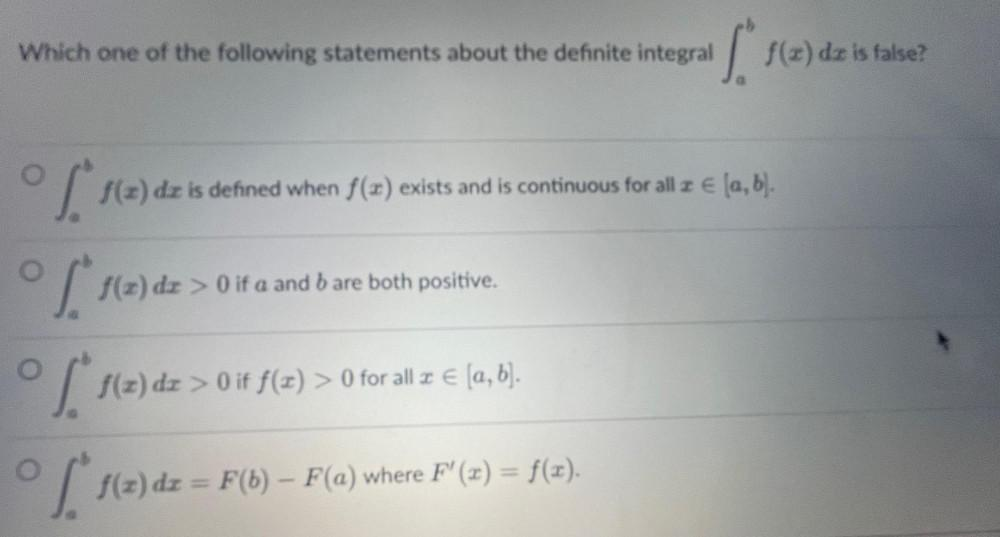Question:

# Which one of the following statements about the definite integral ᵇ ∫a f(x)dx is false? a) ᵇ∫a f(x)dx is defined when f(a) exists and is continuous for all x∈ (a, b). b) ᵇ∫a f(x)dx> 0 if a and b are bWhich one of the following statements about the definite integral ᵇ ∫a f(x)dx is false? a) ᵇ∫a f(x)dx is defined when f(a) exists and is continuous for all x∈ (a, b). b) ᵇ∫a f(x)dx> 0 if a and b are both positive. c) ᵇ∫a f(x)dx> 0 if f(x) > 0 for all z € [a, b]. d) ᵇ∫a f(x)dx = F(b) – F(a) where F'(x) = f(x).